Data Structures and Algorithms with Object-Oriented Design Patterns in C## Projects

1. Write a non-recursive method to compute the factorial of n according to Equation. Calculate the running time predicted by the detailed model given in Sectionand the simplified model given in Section.
2. Write a non-recursive method to compute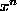according to Equation. Calculate the running time predicted by the detailed model given in Sectionand the simplified model given in Section.
3.   Write a program that determines the values of the timing parameters of the detailed model (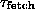,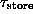,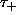,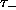,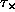,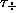,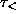,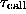,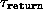,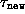, and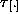) for the machine on which it is run.
4. Using the program written for Project, determine the timing parameters of the detailed model for your computer. Then, measure the actual running times of Programs,andand compare the measured results with those predicted by Equations,and(respectively).
5. Given a sequence of n integers,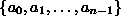, and a small positive integer k, write an algorithm to compute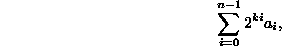without multiplication. Hint: Use Horner's rule and bitwise shifts.

6. Verify Equationexperimentally as follows: Generate a large number of random sequences of length n,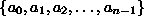. For each sequence, test the hypothesis that the probability that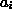is larger than all its predecessors in the sequence is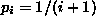. (For a good source of random numbers, see Section).Copyright © 2001 by Bruno R. Preiss, P.Eng. All rights reserved.